# Chemistry Test 5 - Chemical Kinetics, Surface Chemistry, Aldehyde, Ketones, P-Block (Carbon And Boron Family)

## 25 Questions MCQ Test Mock Test Series for JEE Main & Advanced 2022 | Chemistry Test 5 - Chemical Kinetics, Surface Chemistry, Aldehyde, Ketones, P-Block (Carbon And Boron Family)

Description
Attempt Chemistry Test 5 - Chemical Kinetics, Surface Chemistry, Aldehyde, Ketones, P-Block (Carbon And Boron Family) | 25 questions in 60 minutes | Mock test for JEE preparation | Free important questions MCQ to study Mock Test Series for JEE Main & Advanced 2022 for JEE Exam | Download free PDF with solutions
QUESTION: 1

Solution:
QUESTION: 2

Solution:
QUESTION: 3

### When propyne is treated with aqueous H2SO4   in the presence of  HgSO4  , the major product obtained is

Solution:
QUESTION: 4
The formation of cyanohdyrin from a ketone is an example of
Solution:
QUESTION: 5

An organic compound, C3H6O  does not give a precipitate with 2, 4-dinitrophenylhydrazine reagent and does not react with metallic sodium. It could be

Solution:
QUESTION: 6
The end product ‘C’ in the following sequence of chemical reactions is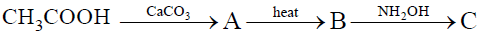Solution:
QUESTION: 7
The reaction in which sodium cyanide is used is
Solution:
QUESTION: 8

Aldol condensation will not take place in

Solution:
QUESTION: 9
Calcium formate on dry heating yields
Solution:
QUESTION: 10

Reduction of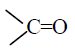to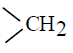can be carried out with

Solution:
QUESTION: 11

Identify the compounds A and B in the following reaction sequence.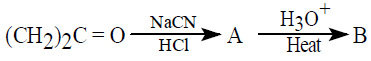Solution:
QUESTION: 12

Which of the following has the most acide hydrogen

Solution:

2, 4-hexanedione (a 1, 3-diketone) has the most acidic hydrogen. This is because the carbanion left after the removal of H^+ is resonance stabilised in this case.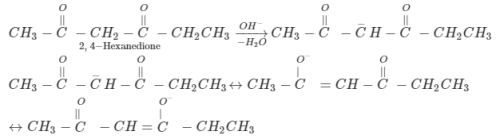QUESTION: 13

An organic compound ‘A’ has the molecular formula C3H6O   It undergoes iodoform test. when saturated with HCl, it gives ‘B’of molecular formula C9H14O  ‘A’ and ‘B’ respectively are

Solution:
QUESTION: 14
Acetone is mixed with bleaching powder to give
Solution:
QUESTION: 15
The dipole moment is the highest for
Solution:
QUESTION: 16

With which of the following reagents, carbonyl compound shows addition cum elimination reaction ?

Solution:
QUESTION: 17
Self-condensation of ethyl acetate in presence of sodium ethoxide yields
Solution:
QUESTION: 18

Acetone on distillation with conc. H2SO4  forms

Solution:
QUESTION: 19
The rate of a gaseous reaction is given by the expression k [A][B]. If the volume of the reaction vessel is suddenly reduced to 1/4th of the initial volume, the reaction rate relting to original rate will be
Solution: R=k[A][B]
[Concentration]=moles/volume
moles is constant and volume is reduced to V/4
R=k[n1/V][n2/V]
R2=k[n1/(V/4)][n2/(V/4)]
R2=16R1
R2/R1=16
QUESTION: 20
For an endothermic reaction, where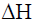represents the enthalpy of the reaction in k.J./mol, the minimum value for the energy of activation will be
Solution: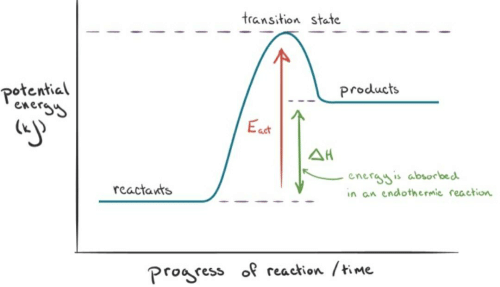*Answer can only contain numeric values
QUESTION: 21

At a certain temperature, the half-life periods for the catalytic decomposition of NH3 were found to be as follows :-
Pressue (mm Hg)         50        100      200
Half-life period (hrs.)   3.52     1.76     0.88
What will be the pressure when the half-life period is 2.5 hours.

Solution: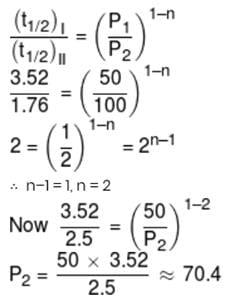*Answer can only contain numeric values
QUESTION: 22

How many of the following elements form amphoteric oxides?
Si, P, N, B, Sn, Pb, O

Solution:

Sn, Pb

*Answer can only contain numeric values
QUESTION: 23

One gm of activated carbon has surface area of 1000 m2. Considering complete coverage as well as mono molecular adsorption, how much ammonia at 1 atm and 273 K would be adsorbed on the surface of 44/7 g carbon if radius of ammonia molecule is 10–8 cm (NA = 6 × 1023)

Solution:
*Answer can only contain numeric values
QUESTION: 24

1gm of dry green algae absorbs 5 moles of CO2 per hour by photo synthesis. If fixed carbon atoms were all stored in the form of starch (C6H12O6)n  after photo synthesis then calculate time required ( in sec.) to double the weight of algae.

Solution: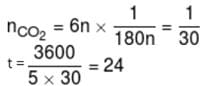*Answer can only contain numeric values
QUESTION: 25

In the electronic configuration of Zn (Z = 30) find the total number of occupied orbitals which do not possess any nodal plane :-

Solution:Use Code STAYHOME200 and get INR 200 additional OFF Use Coupon Code